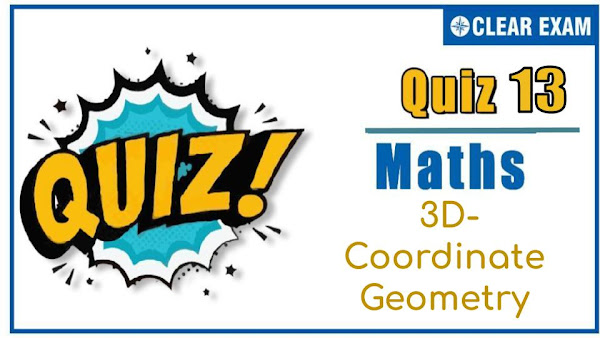## [LATEST]$type=sticky$show=home$rm=0$va=0$count=4$va=0

As per analysis for previous years, it has been observed that students preparing for JEE MAINS find Mathematics out of all the sections to be complex to handle and the majority of them are not able to comprehend the reason behind it. This problem arises especially because these aspirants appearing for the examination are more inclined to have a keen interest in Mathematics due to their ENGINEERING background. Furthermore, sections such as Mathematics are dominantly based on theories, laws, numerical in comparison to a section of Engineering which is more of fact-based, Physics, and includes substantial explanations. By using the table given below, you easily and directly access to the topics and respective links of MCQs. Moreover, to make learning smooth and efficient, all the questions come with their supportive solutions to make utilization of time even more productive. Students will be covered for all their studies as the topics are available from basics to even the most advanced..
3D Coordinate Geometry
Q1. The shortest distance between the lines 1+x=2y=-12z and x=y+2=6z-6 is
•  1
•  2
•  3
•  4
Q2.The equation of the plane passing through (2, 3, 4) and parallel to the plane 5x-6y+7z=3 is
•  5x-6y+7z+20=0
•  5x-6y+7z-20=0
•  -5x+6y-7z+3=0
•  5x-6y+7z+3=0
Solution
(b) Equation of any plane passing through (2, 3, 4) is
A(x-2)+B(y-3)+C(z-4)=0 …(i)
Plane (i) is parallel to 5x-6y+7z=3
∴ DR’s of this plane is same as the Eq. (i)
ie,A=5,B=-6,C=7
∴5(x-2)-6(y-3)+7(z-4)=0

∴ 5x-6y+7z-20=0 is the required plane

Q3.  The equation of the plane perpendicular to the line $$\frac{(x-1)}{1}=\frac{(y-2)}{(-1)}=\frac{(z+1)}{2}$$and passing through the point (2, 3, 1), is
•   $\stackrel{\to }{\mathrm{r.}}$(i ̂+j ̂+2k)=1
•  $\stackrel{\to }{\mathrm{r.}}$(i ̂-j ̂+2k)=1
•  $\stackrel{\to }{\mathrm{r.}}$(i ̂-j ̂+2k)=7
•  $\stackrel{\to }{\mathrm{r.}}$(i ̂+j ̂-2k)=10
Solution
Not available

Q4. A line makes the same angle θ with each of the x and z axes. If the angle β, which it makes with y-axis, is such that sin2θ, then cos2θ equals
•  2/3
•  1/5
•  2/5
•  3/5
Solution
(c) Since, l2+m2+n2=1
⟹ cos2 θ+cos2 β+cos2 θ=1
[∴l=cos⁡〖θ,m=cos⁡〖β,given]〗 〗
⟹cos2 θ+cos2 β
¬But sin2 β=3 sin2⁡θ

∴ 3sin2 θ=〖2cos〗2 θ
⟹3=5 cos2 θ
⟹ cos2 θ=3/5

Q5.If a plane meets the coordinate axes at A,B and C such that the centroid of the triangle is (1,2,4), then
the equation of the plane is
•  x+2y+4z=12
•  4x+2y+z=12
•  x+2y+4z=3
•  4x+2y+z=3
Q6. If O is the origin and OP=3 with direction ratios -1, 2, -2, then coordinates of P are
•  (1, 2, 2)
•  (-1,2,-2)
• (-3,6,-9)
•  (-1/3,2/3,-2/3)
Q7.The ratio in which the line joining (2,4,5), (3,5,-4) is divided by the yz-plane is
•   2 : 3
•   3 : 2
•  -2 : 3
•   4 : -3
Q8. The area of triangle whose vertices are (1, 2, 3), (2, 5, -1) and (-1,1,2) is
•   150 sq unit
•   145 sq unit
•  √155/2 sq unit
•   155/2 sq unit
Q9. The radius of the circle of x+2y+2z=15,x2+y2+z2-2y-4z=11 is
•   2
•  √7
•   3
•  √5
Q10. The cartesian equation of the plane perpendicular to the line $$\frac{(x-1)}{2}=\frac{(y-3)}{-1}=\frac{(z-4)}{2}$$ and passing through the origin is
•  2x-y+2z-7=0
•  2x+y+2z=0
•  2x-y+2z=0
• 2x-y-z=0
Solution
(c) It is given that the line
 $$\frac{(x-1)}{2}=\frac{(y-3)}{-1}=\frac{(z-4)}{2}$$
Is perpendicular to the required.
This means that the normal to the plane is parallel to the line.
So, its direction ratios are proportional to 2,-1,2
The plane passes through the origin

Hence, its equation is
2(x-0)-(y-0)+2(z-0)=0⇒2x-y+2z=0## Want to know more

Please fill in the details below:

## Latest IITJEE Articles$type=three$c=3$author=hide$comment=hide$rm=hide$date=hide$snippet=hide ## Latest NEET Articles$type=three$c=3$author=hide$comment=hide$rm=hide$date=hide$snippet=hide

Name

ltr
item
BEST NEET COACHING CENTER | BEST IIT JEE COACHING INSTITUTE | BEST NEET, IIT JEE COACHING INSTITUTE: 3D Coordinate Geometry Quiz-13
3D Coordinate Geometry Quiz-13
https://1.bp.blogspot.com/-EusqeAkORqE/X42U0J1FB5I/AAAAAAAAAW4/CvWcbOFuSNcaOhF_DEloT_yXd5EuyYfcQCLcBGAsYHQ/s600/Untitled%2Bpresentation%2B%252813%2529.jpg
https://1.bp.blogspot.com/-EusqeAkORqE/X42U0J1FB5I/AAAAAAAAAW4/CvWcbOFuSNcaOhF_DEloT_yXd5EuyYfcQCLcBGAsYHQ/s72-c/Untitled%2Bpresentation%2B%252813%2529.jpg
BEST NEET COACHING CENTER | BEST IIT JEE COACHING INSTITUTE | BEST NEET, IIT JEE COACHING INSTITUTE
https://www.cleariitmedical.com/2020/10/3DCoordinateQuiz13.html
https://www.cleariitmedical.com/
https://www.cleariitmedical.com/
https://www.cleariitmedical.com/2020/10/3DCoordinateQuiz13.html
true
7783647550433378923
UTF-8